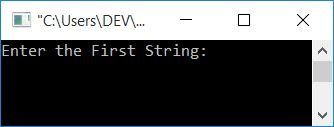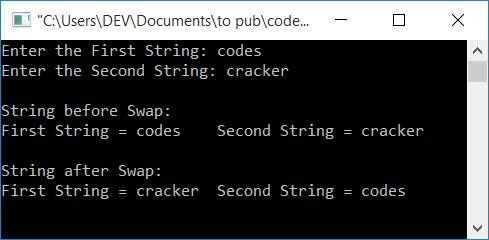# C Program to Swap Two Strings

In this article, you will learn and get code for the swapping of two given strings in C programming using the following approaches:

Let's first create a program that uses a library function. Then later on, you will get the program without any library function to swap two strings.

## Using a Function, Swap Two Strings in C

This program uses the function strcpy(). This function accepts two character arguments. The value of the second argument gets copied to the first one. For example, if there are two character-type variables, say num1 and num2, and let's suppose num2 holds its value as a "codescracker." Then the following statement:

`strcpy(num1, num2);`

copies the value of num2 to num1. Both variables now contain the same value, "codescracker." The function strcpy() is defined in the string.h header file. Now let's move on to the program:

```#include<stdio.h>
#include<conio.h>
#include<string.h>
int main()
{
char str1, str2, temp;
printf("Enter the First String: ");
gets(str1);
printf("Enter the Second String: ");
gets(str2);
printf("\nString before Swap:\n");
printf("First String = %s\tSecond String = %s", str1, str2);
strcpy(temp, str1);
strcpy(str1, str2);
strcpy(str2, temp);
printf("\n\nString after Swap:\n");
printf("First String = %s\tSecond String = %s", str1, str2);
getch();
return 0;
}```

This program was built and runs in the Code::Blocks IDE. Here is its sample run:Now enter the first string, say codes, and then enter the second string, say cracker, to perform the swap operation of the given two strings as shown in the following output:As you can see from the above program, the main code for swapping the two strings is:

```strcpy(temp, str1);
strcpy(str1, str2);
strcpy(str2, temp);```

If the user enters codes and cracker as the first and second string values. That is, str1 is for codes and str2 is for cracker. Then, after executing the first statement,
`strcpy(temp, str1);`
The value of str1 gets copied to temp. Therefore, now temp=codes.

Following the execution of the second statement,
`strcpy(str1, str2);`
The value of str2 gets copied to str1. As a result, now str1=cracker.

Finally, after executing the third statement,
`strcpy(str2, temp);`
The value of temp gets copied to str2. As a result, str2=codes.

As you can see, after executing the three statements given above, str1 holds the value that str2 previously had, and vice versa

## Swap two strings without using library functions in C

This program swaps two given strings without using any type of library function.

```#include<stdio.h>
#include<conio.h>
int main()
{
char str1, str2, temp;
int i=0;
printf("Enter the First String: ");
gets(str1);
printf("Enter the Second String: ");
gets(str2);
printf("\nString before Swap:\n");
printf("First String = %s\tSecond String = %s", str1, str2);
while(str1[i]!='\0')
{
temp[i] = str1[i];
i++;
}
temp[i] = '\0';
i=0;
while(str2[i]!='\0')
{
str1[i] = str2[i];
i++;
}
str1[i] = '\0';
i=0;
while(temp[i]!='\0')
{
str2[i] = temp[i];
i++;
}
str2[i] = '\0';
printf("\n\nString after Swap:\n");
printf("First String = %s\tSecond String = %s", str1, str2);
getch();
return 0;
}```

It will produce the same output as the previous program. In this program, we've first copied all the characters of the first string, str1, to a variable, temp, in a character-by-character manner. Then I copied all of the characters from the second string, str2, to the first string, str1. Finally, I copied all of the characters from temp to the second string, let us call it str2.

Remember to add a null-terminated character ('\0') as the last character to all variables such as str1, str2, and temp.

For instance, if the user types in codes and cracker as two strings, Then code gets initialized to the num1 variable in a way that:

• num1 = c
• num1 = o
• num1 = d
• num1 = e
• num1 = s

The same happened with the second string. Now with the following block of code:

```while(str1[i]!='\0')
{
temp[i] = str1[i];
i++;
}```

Because the initial value of i is 0, all 5 characters of the "num1" variable are initialized one by one to the "temp" variable in the following manner:

• temp[i] = str1[i] or temp = str1 or temp = c
• temp[i] = str1[i] or temp = str1 or temp = o
• temp[i] = str1[i] or temp = str1 or temp = d
• Similarly temp = e and temp = s
• And then temp = \0

"temp = codes." Again, set the value of i to 0 and repeat for the next one. In this way, the swapping of two strings gets performed.

#### The same program in different languages

C Quiz

« Previous Program Next Program »